# Samacheer Kalvi 12th Maths Solutions Chapter 2 Complex Numbers Ex 2.8

## Tamilnadu Samacheer Kalvi 12th Maths Solutions Chapter 2 Complex Numbers Ex 2.8

Question 1.
If to ω ≠ 1 is a cube root of unity, then show that $$\frac{a+b \omega+c \omega^{2}}{b+c \omega+a \omega^{2}}+\frac{a+b \omega+c \omega^{2}}{c+a \omega+b \omega^{2}}=1$$
Solution:
Since ω is a cube root of unity, we have ω3 = 1 and 1 + ω + ω2 = 0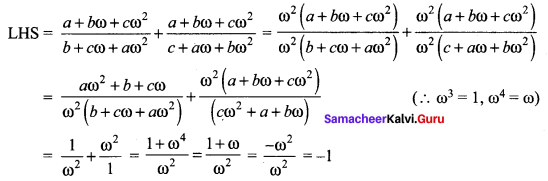Question 2.
Show that $$\left(\frac{\sqrt{3}}{2}+\frac{i}{2}\right)^{5}+\left(\frac{\sqrt{3}}{2}-\frac{i}{2}\right)^{5}=-\sqrt{3}$$
Solution: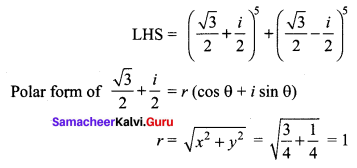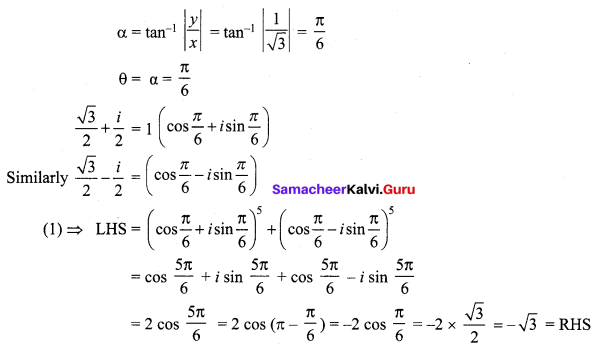Question 3.
Find the value of $$\left(\frac{1+\sin \frac{\pi}{10}+i \cos \frac{\pi}{10}}{1+\sin \frac{\pi}{10}-i \cos \frac{\pi}{10}}\right)^{10}$$
Solution: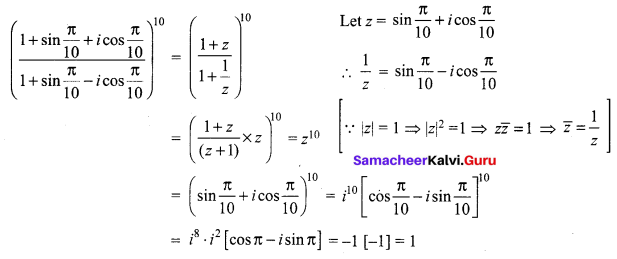Question 4.
If 2 cos α = x + $$\frac{1}{x}$$ and 2 cos β = y + $$\frac{1}{y}$$, show that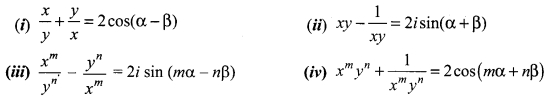Solution:
(i) 2 cos α = x + $$\frac{1}{x}$$
⇒ 2 cos α = $$\frac{x^{2}+1}{x}$$
⇒ 2x cos α = x2 + 1
⇒ x2 – 2x cos α + 1 = 0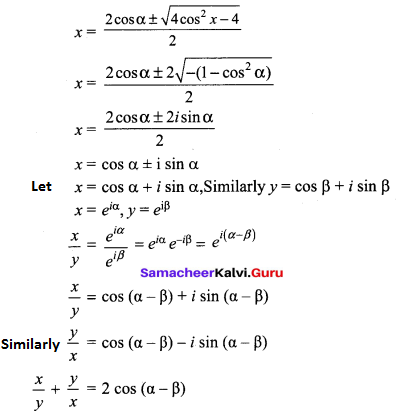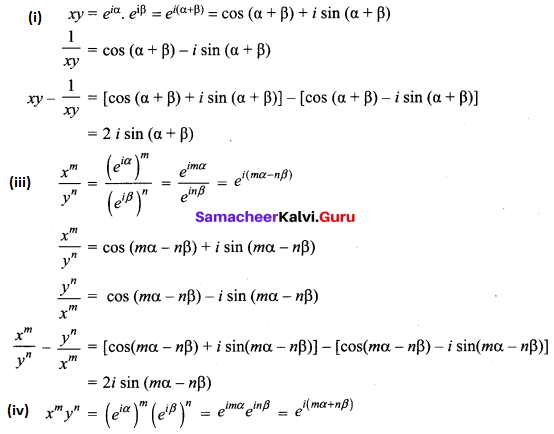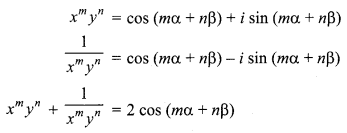Question 5.
Solve the equation z3 + 27 = 0
Solution:
z3 + 27 = 0
⇒ z3 = -27
⇒ z3 = 33(-1)
⇒ z = 3(-1)1/3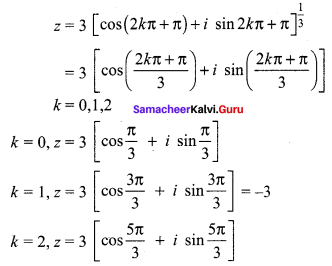Question 6.
If ω ≠ 1 is a cube root of unity, show that the roots of the equation (z – 1)3 + 8 = 0 are -1, 1 – 2ω, 1 – 2ω2
Solution:
(z – 1)3 + 8 = 0
⇒ (z – 1 )3 = -8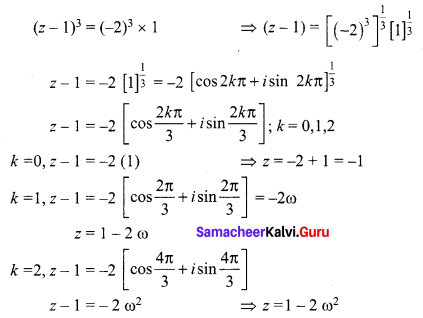The roots are -1, 1 – 2ω, 1 – 2ω2

Question 7.
Find the value of $$\sum_{k=1}^{8}\left(\cos \frac{2 k \pi}{9}+i \sin \frac{2 k \pi}{9}\right)$$
Solution:
$$\sum_{k=1}^{8}\left(\cos \frac{2 k \pi}{9}+i \sin \frac{2 k \pi}{9}\right)$$
We know that 9th roots of unit are 1, ω, ω2, ……., ω8
Sum of the roots:
1 + ω + ω2 + …. + ω8 = 0 ⇒ ω + ω2 + ω3 + …… + ω8 = -1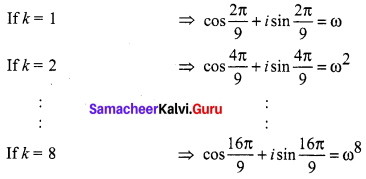The sum of all the terms $$\sum_{k=1}^{8}\left(\cos \frac{2 k \pi}{9}+i \sin \frac{2 k \pi}{9}\right)$$ = -1

Question 8.
If ω ≠ 1 is a cube root of unity, show that
(i) (1 – ω + ω2)6 + (1 + ω – ω2)6 = 128
(ii) (1 + ω)(1 + ω2)(1 + ω4)(1 + ω8)……(1 + ω2n) = 1
Solution:
(i) ω is a cube root of unity ω3 = 1; 1 + ω + ω2 = 0
(1 – ω + ω2)6 + (1 + ω – ω2)6
= (-ω – ω)6 + (-ω2 – ω2)6
= (-2ω)6 + (-2ω2)6
= (-2)66 + ω12)
= (64)(1 + 1)
= 128
(ii) (1 + ω)(1 + ω2)(1 + ω4)(1 + ω8) …… (1 + ω2n)
= (1 + ω)(1 + ω2)(1 + ω4)(1 + ω8) ……. 2n factors
= (-ω2)(-ω)(-ω2)(-ω) …… 2n factors
= ω3. ω3
= 1

Question 9.
If z = 2 – 2i, find the rotation of z by θ radians in the counter clockwise direction about the origin when
(i) θ = $$\frac{\pi}{3}$$
(ii) θ = $$\frac{2 \pi}{3}$$
(iii) θ = $$\frac{3 \pi}{2}$$
Solution:
(i) z = 2 – 2i = 2 (1 – i) = r(cos θ + i sin θ)
$$r=\sqrt{x^{2}+y^{2}}=2 \sqrt{1+1}=2 \sqrt{2}$$
$$\alpha=\tan ^{-1}=\left|\frac{y}{x}\right|=\tan ^{-1}|1|=\frac{\pi}{4}$$
(1 – i) lies in IV quadrant
θ = -α = $$-\frac{\pi}{4}$$
$$\Rightarrow z=2 \sqrt{2}\left[\cos \left(\frac{-\pi}{4}\right)+i \sin \left(\frac{-\pi}{4}\right)\right]$$
z is rotated by θ = $$\frac{\pi}{3}$$ in the counter clock wise direction.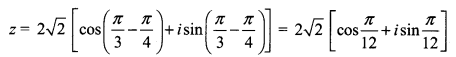(ii) z is rotated by θ = $$\frac{2 \pi}{3}$$ in the counter clockwise direction.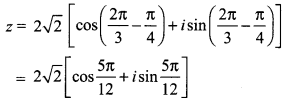(iii) z is rotated by θ = $$\frac{3 \pi}{2}$$ in the counter clockwise direction.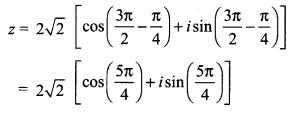Question 10.
Prove that the values of $$\sqrt{-1} \text { are } \pm \frac{1}{\sqrt{2}}(1 \pm i)$$
Solution: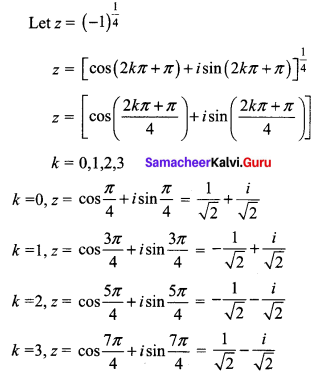The roots are $$\pm \frac{1}{\sqrt{2}}(1 \pm i)$$

### Samacheer Kalvi 12th Maths Solutions Chapter 2 Complex Numbers Ex 2.8 Additional Problems

Question 1.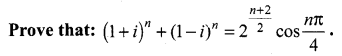Solution: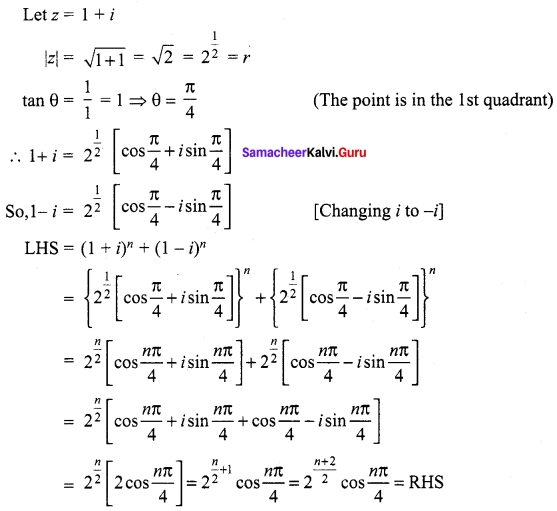Question 2.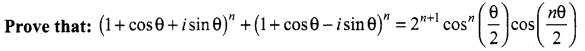Solution: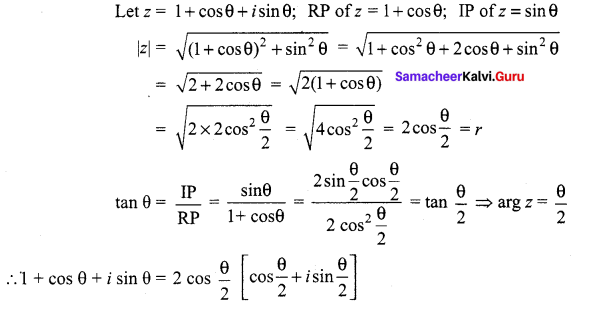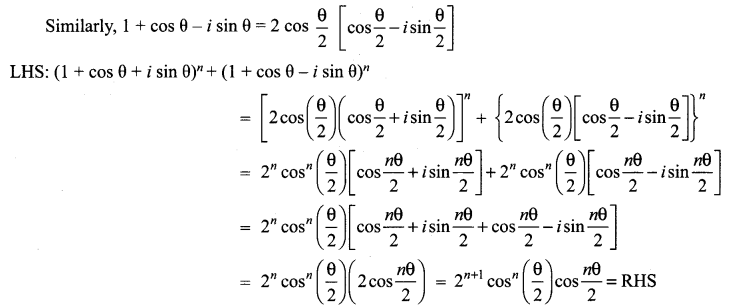Question 3.
Prove that: (1 + i)4n and (1 + i)4n + 2 are real and purely imaginary respectively.
Solution:
Let z = 1 + i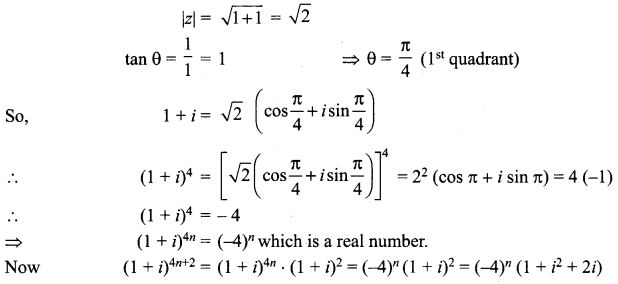= 2i (-1)n which is purely imaginary.Question 4.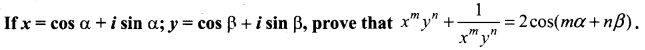Solution: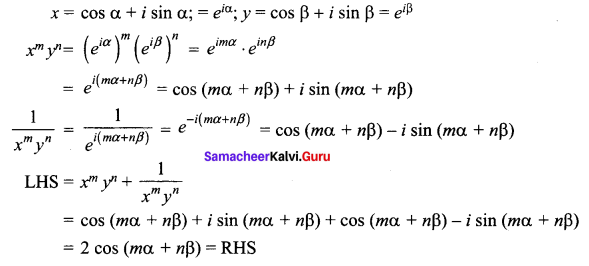Question 5.
If a = cos 2α + i sin 2α, b = cos 2β + i sin 2β and c = cos 2γ + i sin 2γ, prove that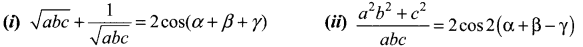Solution: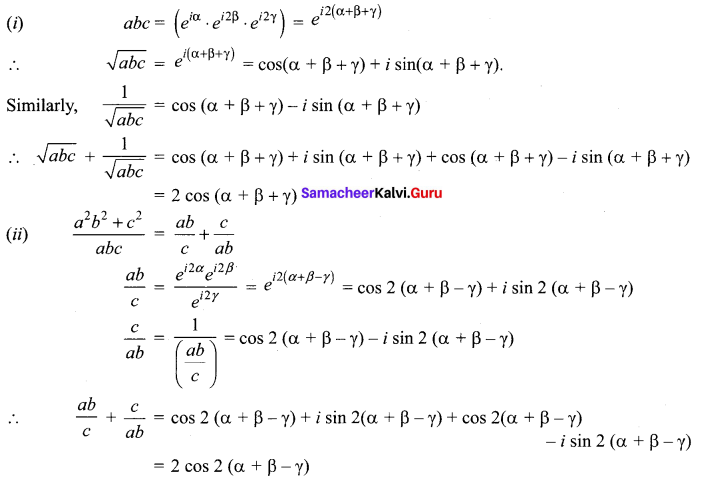Question 6.
Solve: x4 + 4 = 0
Solution: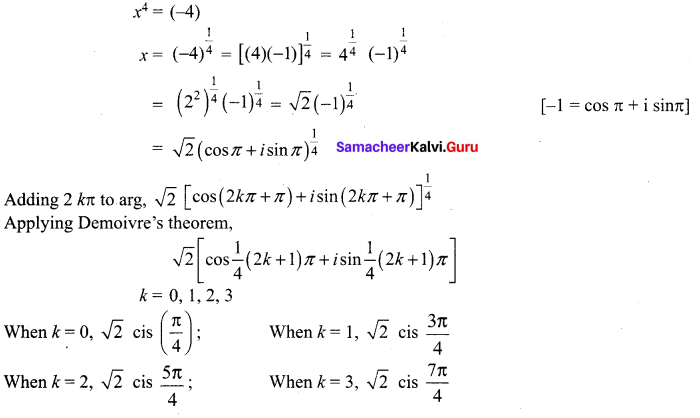Question 7.
If x = a + b, y = aω + bω2, z = aω2 + bω, show that
(i) xyz = a3 + b3
(ii) x3 + y3 + z3 = 3(a3 + b3)
Solution:
(i) x = a + A; y = aω + bω2, z = aω2 + bω
Now xyz = (a + b) (aω + bω2) (abω2 + bω) = (a+A) [aω3 + abω2 + abω + b2ω3]
= (a + b) (a2 – ab + b2) = a3 + b3
xyz = a3 + b3

(ii) x = a + b, y = aω + bω2, z = aω2 + bω
x + y + z = (a + aω + aω2) + (b + bω2 + bω)
= a (1 + ω + ω2) + b (1 + ω + ω2) = a(0) + b(0) = 0
Now x + y + z = 0 ⇒ x3 + y3 + z3 = 3xyz
Here xyz = a3 + b3
∴ x3 + y3 + z3 = 3 (a3 + b3)